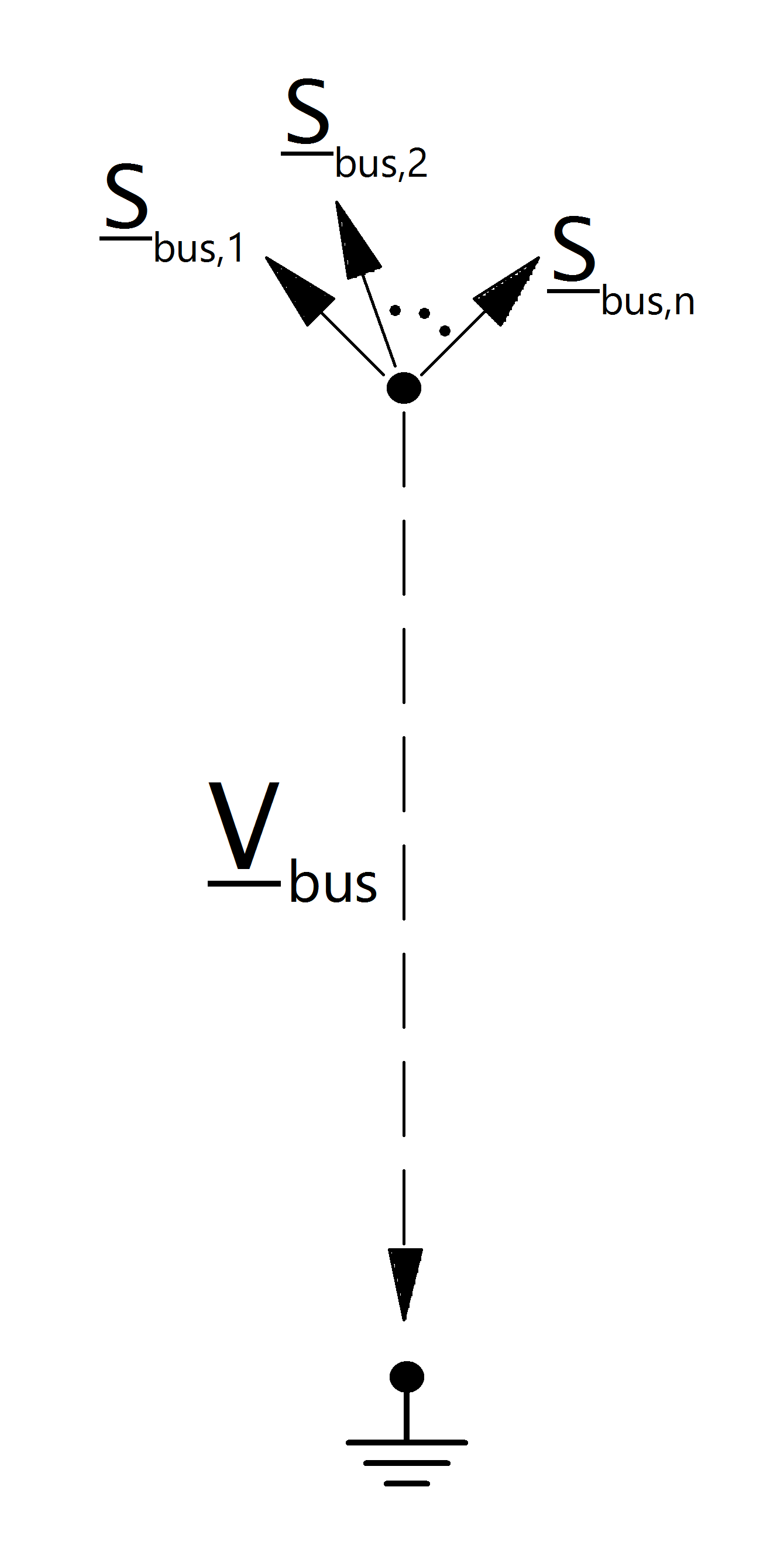# Bus

## Create Function

pandapower.create_bus(net, vn_kv, name=None, index=None, geodata=None, type='b', zone=None, in_service=True, max_vm_pu=nan, min_vm_pu=nan, coords=None, **kwargs)

Adds one bus in table net[“bus”].

Busses are the nodes of the network that all other elements connect to.

INPUT:

net (pandapowerNet) - The pandapower network in which the element is created

vn_kv (float) - The grid voltage level.

OPTIONAL:

name (string, default None) - the name for this bus

index (int, default None) - Force a specified ID if it is available. If None, the index one higher than the highest already existing index is selected.

geodata ((x,y)-tuple, default None) - coordinates used for plotting

type (string, default “b”) - Type of the bus. “n” - node, “b” - busbar, “m” - muff

zone (string, None) - grid region

in_service (boolean) - True for in_service or False for out of service

max_vm_pu (float, NAN) - Maximum bus voltage in p.u. - necessary for OPF

min_vm_pu (float, NAN) - Minimum bus voltage in p.u. - necessary for OPF

coords (list (len=2) of tuples (len=2), default None) - busbar coordinates to plot the bus with multiple points. coords is typically a list of tuples (start and endpoint of the busbar) - Example: [(x1, y1), (x2, y2)]

OUTPUT:

index (int) - The unique ID of the created element

EXAMPLE:

create_bus(net, name = “bus1”)

## Input Parameters

net.bus

 Parameter Datatype Value Range Explanation name string name of the bus vn_kv* float $$>$$ 0 rated voltage of the bus [kV] type string naming conventions: “n” - node “b” - busbar “m” - muff type variable to classify buses zone string can be used to group buses, for example network groups / regions max_vm_pu** float $$>$$ 0 Maximum voltage min_vm_pu** float $$>$$ 0 Minimum voltage in_service* boolean True / False specifies if the bus is in service.

*necessary for executing a power flow calculation
**optimal power flow parameter

Note

Bus voltage limits can not be set for slack buses and will be ignored by the optimal power flow.

net.bus_geodata

 Parameter Datatype Explanation x float x coordinate of bus location y float y coordinate of bus location

## Electric Model## Result Parameters

net.res_bus

 Parameter Datatype Explanation vm_pu float voltage magnitude [p.u] va_degree float voltage angle [degree] p_mw float resulting active power demand [MW] q_mvar float resulting reactive power demand [Mvar]

The power flow bus results are defined as:

\begin{align*} vm\_pu &= \lvert \underline{V}_{bus} \rvert \\ va\_degree &= \angle \underline{V}_{bus} \\ p\_mw &= Re(\sum_{n=1}^N \underline{S}_{bus, n}) \\ q\_mvar &= Im(\sum_{n=1}^N \underline{S}_{bus, n}) \end{align*}

net.res_bus_3ph

 Parameter Datatype Explanation vm_a_pu float voltage magnitude:Phase A [p.u] va_a_degree float voltage angle:Phase A [degree] vm_b_pu float voltage magnitude:Phase B [p.u] va_b_degree float voltage angle:Phase B [degree] vm_c_pu float voltage magnitude:Phase C [p.u] va_c_degree float voltage angle:Phase C [degree] p_a_mw float resulting active power demand:Phase A [MW] q_a_mvar float resulting reactive power demand:Phase A [Mvar] p_b_mw float resulting active power demand:Phase B [MW] q_b_mvar float resulting reactive power demand:Phase B [Mvar] p_c_mw float resulting active power demand:Phase C [MW] q_c_mvar float resulting reactive power demand:Phase C [Mvar] unbalance_percent float unbalance in percent defined as the ratio of V0 and V1 according to IEC 62749

The power flow bus results are defined as:

\begin{align*} vm\_pu_{phase} &= \lvert \underline{V_{phase}}_{bus} \rvert \\ va\_degree_{phase} &= \angle \underline{V_{phase}}_{bus} \\ p\_mw_{phase} &= Re(\sum_{n=1}^N \underline{S_{phase}}_{bus, n}) \\ q\_mvar_{phase} &= Im(\sum_{n=1}^N \underline{S_{phase}}_{bus, n}) \end{align*}

net.res_bus_est

The state estimation results are put into net.res_bus_est with the same definition as in net.res_bus.

 Parameter Datatype Explanation vm_pu float voltage magnitude [p.u] va_degree float voltage angle [degree] p_mw float resulting active power demand [MW] q_mvar float resulting reactive power demand [Mvar]

Note

Bus power values are given in the consumer system. Therefore a bus with positive p_mw value consumes power while a bus with negative active power supplies power.

net.res_bus_sc

The short-circuit (SC) results are put into net.res_bus_sc with following definitions:

 Parameter Datatype Explanation ikss_ka float initial short-circuit current value [kA] skss_mw float initial short-circuit power [MW] ip_ka float peak value of the short-circuit current [kA] ith_ka float equivalent thermal short-circuit current [kA] rk_ohm float resistive part of equiv. (positive/negative sequence) SC impedance [Ohm] xk_ohm float reactive part of equiv. (positive/negative sequence) SC impedance [Ohm] rk0_ohm float resistive part of equiv. (zero sequence) SC impedance [Ohm] xk0_ohm float reactive part of equiv. (zero sequence) SC impedance [Ohm]